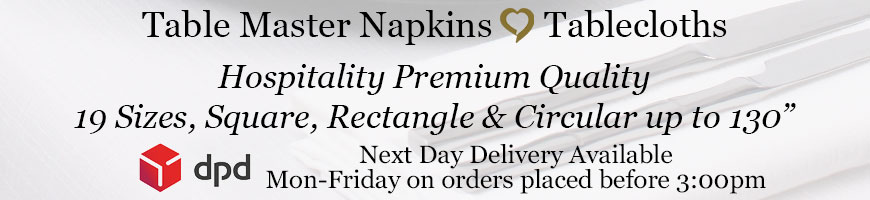# Measure your table size, then calculate the correct size tablecloth required by including the drop down the table

The Drop is how much the tablecloth will drop around the table

The Clearance is the distance from the bottom of the tablecloth to the floor

* A standard height of a table is 29" - 30"

Find your table size in the left column

View the options of tablecloth sizes for your table by using the horizontal columnExample - Rectangular Table -  Width & Length Of The Table Top

Rectangular table 2ft 6"wide x 6ft length  =  30" x 72" This is a standard trestle table size

A tablecloth size of 70" x 108" will give a drop of 20" down each side and 18" down each end

A tablecloth size of 83" x 126" will give a drop of 27" down each side and 27" down each end [Full table coverage]

* A standard height of a table is 29" - 30"Calculation Method For Circular Tablecloth Size

(Tablecloth Size Diameter) Minus (Diameter Of The Table Top Surface) Then Divide By 2 = The Drop Around The Table

E.g. Tablecloth size diameter 130" (Minus) diameter of the table top surface 72" = 58"

Then 58" divide by 2 = 29" tablecloth drop around the table

Example - Circular Round Table 72" Diameter Of The Table Top Surface

A tablecloth size of 88" will give a drop around the table of 8" around the table

A tablecloth size of 108" will give a drop around the table of 18" around the table

A tablecloth size of 118" will give a drop around the table of 23" around the table

A tablecloth size of 130" will give a drop around the table of 29" around the table [Full coverage]

* A standard height of a table is 29"- 30"

Need any help on what is the correct size you require?

Measure your table size -  width and length or diameter

Send the details by using the Enquires & Sample Request Form

We Love To Talk call us on Free Phone 0800 612 1379